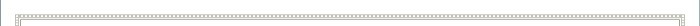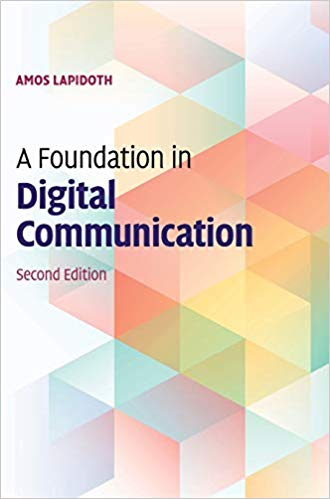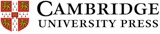A Foundation in Digital CommunicationTitle: A Foundation in Digital Communications Author: Amos Lapidoth Edition: Second edition ISBN 13: 9781107177321 Publisher: Cambridge University Press Publish Date: February 2017 Binding: Hardcover, 916 pages Weight: 1.92 kg Exercises: 501 Figures: 93Amos Lapidoth

This intuitive but rigorous introduction derives the core results and engineering schemes of digital communication from first principles. Theory, rather than industry standards, motivates the engineering approaches, and key results are stated with all the required assumptions.

The book emphasizes the geometric view, opening with the inner product, the matched filter for its computation, Parseval's theorem, the sampling theorem as an orthonormal expansion, the isometry between passband signals and their baseband representation, and the spectral-efficiency optimality of quadrature amplitude modulation (QAM). Subsequent chapters address noise, with a comprehensive study of hypothesis testing, Gaussian stochastic processes, the sufficiency of the matched filter outputs, and some coding theory.

New is a treatment of white noise without generalized functions and a presentation of the power spectral density without artificial random jitters and random phases in the analysis of QAM.

This second edition includes entirely new chapters on the radar problem (with Lyapunov's theorem) and intersymbol interference channels, new discussion of the baseband representation of passband noise, and a simpler, more geometric derivation of the optimal receiver for the additive white Gaussian noise channel. Other key topics covered include the geometry of the space of energy-limited signals, the isometry properties of the Fourier transform, and complex sampling.

Including over 500 homework problems and all the necessary mathematical background, this is the ideal text for one- or two-semester graduate courses on digital communications and courses on stochastic processes and detection theory. Solutions to problems and video lectures are available online.

Contents

 Preface to the second edition Preface to the first edition Acknowledgments for the second edition Acknowledgments for the first edition 1. Some essential notation 2. Signals, integrals, and sets of measure zero 3. The inner product 4. The space L2 of energy-limited signals 5. Convolutions and filters 6. The frequency response of filters and bandlimited signals 7. Passband signals and their representation 8. Complete orthonormal systems and the sampling theorem 9. Sampling real passband signals 10. Mapping bits to waveforms 11. Nyquist's criterion 12. Stochastic processes: definition 13. Stationary discrete-time stochastic processes 14. Energy and power in PAM 15. Operational power spectral density 16. Quadrature amplitude modulation 17. Complex random variables and processes 18. Energy, power, and PSD in QAM 19. The univariate Gaussian distribution 20. Binary hypothesis testing 21. Multi-hypothesis testing 22. Sufficient statistics 23. The multivariate Gaussian distribution 24. Complex Gaussians and circular symmetry 25. Continuous-time stochastic processes 26. Detection in white Gaussian noise 27. Noncoherent detection and nuisance parameters 28. Detecting PAM and QAM signals in white Gaussian noise 29. Linear binary block codes with antipodal signaling 30. The radar problem 31. A glimpse at discrete-time signal processing 32. Intersymbol interference App. A: On the Fourier series App. B: On the discrete-time Fourier transform App. C: Positive definite functions App. D: The baseband representation of passband stochastic processes Bibliography Theorems referenced by name Abbreviations List of symbols Index